# Six Sigma / SPC

Six Sigma is a development on SPC which attempts to broaden the usage of traditional SPC (Statistical Process Control) and incorporate elements of TQM, but both Six Sigma and SPC can be viewed as another TQM tool.

#### Process Sigma Calculation

Defects Per Million Opportunities (DPMO) = (Total Defects / Total Opportunities) * 1,000,000

Defects (%) = (Total Defects / Total Opportunities)* 100%

Yield (%) = 100 - %Defects

#### Using Excel to Calculate Sigma

Process Sigma (type this formula into Excel):

=NORMSINV(1-(total defects / total opportunities))+1.5

Be sure to include the Equals (=) sign. This will give you your process sigma (or sigma capability) assuming the 1.5 sigma shift.

For example if you type this into Excel,

=NORMSINV(1-100/1000000)+1.5

you will get 5.22 for your Process Sigma.

How To Determine Sample Size, Determining Sample Size

In order to prove that a process has been improved, you must measure the process capability before and after improvements are implemented. This allows you to quantify the process improvement (e.g. defect reduction or productivity increase) and translate the effects into an estimated financial result something business leaders can understand and appreciate. If data is not readily available for the process, how many members of the population should be selected to ensure that the population is properly represented? If data has been collected, how do you determine if you have enough data?

Determining sample size is a very important issue because samples that are too large may waste time, resources and money, while samples that are too small may lead to inaccurate results. In many cases, we can easily determine the minimum sample size needed to estimate a process parameter, such as the population mean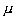When sample data is collected and the sample meanis calculated, that sample mean is typically different from the population meanThis difference between the sample and population means can be thought of as an error. The margin of error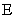is the maximum difference between the observed sample meanand the true value of the population mean: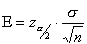where: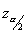is known as the critical value, the positivevalue that is at the vertical boundary for the area of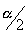in the right tail of the standard normal distribution.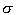is the population standard deviation.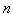is the sample size.Rearranging this formula, we can solve for the sample size necessary to produce results accurate to a specified confidence and margin of error.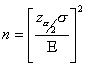This formula can be used when you knowand want to determine the sample size necessary to establish, with a confidence of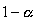, the mean valueto within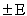. You can still use this formula if you don’t know your population standard deviationand you have a small sample size. Although it’s unlikely that you knowwhen the population mean is not known, you may be able to determinefrom a similar process or from a pilot test/simulation.

Example Problem
We would like to start an Internet Service Provider (ISP) and need to estimate the average Internet usage of households in one week for our business plan and model. How many households must we randomly select to be 95% sure that the sample mean is within 1 minute of the population mean. Assume that a previous survey of household usage has shown= 6.95 minutes.

Solution
We are solving for the sample size.

A 95% degree confidence corresponds to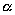= 0.05. Each of the shaded tails in the following figure has an area of= 0.025. The region to the left ofand to the right of= 0 is 0.5 - 0.025, or 0.475. In the Table of the Standard Normal () Distribution, an area of 0.475 corresponds to avalue of 1.96. The critical value is therefore= 1.96.The margin of error= 1 and the standard deviation= 6.95. Using the formula for sample size, we can calculate: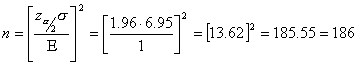So we will need to sample at least 186 (rounded up) randomly selected households. With this sample we will be 95% confident that the sample meanwill be within 1 minute of the true population of Internet usage.

#### Sample Size

By definition, a sample of size n is random if the probability of selecting the sample is the same as the probability of selecting every other sample of size n. If the sample is not random, a bias in introduced which causes a statistical sampling or testing error by systematically favoring some outcomes over others. It is the responsibility of the Quality professional to ensure that samples are random, unbiased and representative of the population.

Let's examine three examples from manufacturing, transaction and ebusiness life that require sampling to ensure process capability:

1. Parts on a manufacturing conveyor line going from one station to the next need to be examined to ensure proper tolerancing.

2. Statements being stuffed into envelopes and then sealed by an automatic machine need to be verified that they are completely sealed.

3. Users visiting your Internet site and clicking through your product catalog should be polled about their online experience.

In these three cases we would like to select a random sample of parts, envelopes and users from a population of 1000 parts, envelopes and users that are produced, sealed or visit the site daily. Let's assume a 95% confidence level, 15% margin of error and population size of 1000. The sample size needed to represent the population is 41. In each of the three cases, there will be significant bias if we were to select the first 41 of the 1000 for that day. That would be convenience sampling and the 'early birds' of each of the processes may not represent the population very well. We cannot select the parts, envelopes or users that we think are appropriate either, as this would introduce serious problems.

How do we decide which parts, envelopes and users to select for our sampling? With a population size of 1000, we could randomly select 41 numbers between 1 and 1000. Where could we get the numbers? They could be generated by a computer program such as Microsoft Excel. For instance, in Excel you would use the following cell formula to derive the first random number of the 41 needed:

=RANDBETWEEN(bottom,top)

where bottom is the smallest integer RANDBETWEEN will return (in this case 1) and top is the largest integer RANDBETWEEN will return (in this case 1000). If this function is not available, you may need to install the Analysis ToolPak by selecting it the Add-Ins command on the Tools menu.

Remember- Users visiting your Internet site always have a choice to close the window if they prefer not to take your survey. Ensure that your sample size is the total number of users you randomly selected minus the number of users that refuse to provide feedback.

One final note on the sample: In the case of the parts and envelopes, they have no choice but to be sampled if you select them. Users visiting your site, on the other hand, always have a choice to close the window if they prefer not to take your survey. Ensure that your sample size is the total number of users you randomly selected minus the number of users that refuse to provide feedback, this will give you an unbiased method for obtaining a random sample.

#### Small (<30) Sample Size Calculation

The formula for the sample size necessary to produce results accurate to a specified confidence and margin of error is:where:is known as the critical value, the positivevalue that is at the vertical boundary for the area ofin the right tail of the standard normal distribution.is the population standard deviation.is the sample size.

This formula can be used when you knowand want to determine the sample size necessary to establish, with a confidence of, the mean valueto within.

As a general rule of thumb, if your sample sizeis greater than 30, you can replaceby the sample standard deviation s.

If your sample size is less than or equal to 30, the population must be normally distributed and you must know the population standard deviationin order to use the formula above. An alternate solution method is to use the Student Distribution developed by William Gosset.

#### How to Calculate Process Sigma

Consider the power company example from the previous page: A power company measures their performance in uptime of available power to their grid. Here is the 5 step process to calculate your process sigma.

#### Step 1: Define Your Opportunities

An opportunity is the lowest defect noticeable by a customer. This definition, of course, is debatable within the Six Sigma community. Here's a useful snippet from the forum discussing this point:

"Typically, most products (and services) have more than one opportunity of going wrong. For example, it is estimated than in electronics assembly a diode could have the following opportunities for error:
1) Wrong diode and
2) wrong polarity (inserted backwards)

So for each assembly shipped, at least two defect opportunities could be assigned for each diode. Apparently, some manufacturers of large complex equipment with many components prefer to count two opportunities in this case. My point is that this approach dilutes Six Sigma metrics." -Anonymous

Many Six Sigma professionals support the counter point. The pioneer of Six Sigma, Motorola built pagers that did not require testing prior to shipment to the customer. Their process sigma was around six, meaning that only approximately 3.4 pagers out of a million shipped did not function properly when the customer received it. The customer doesn't care if the diode is backwards or is missing, just that the pager works.

Returning to our power company example, an opportunity was defined as a minute of up-time. That was the lowest (shortest) time period that was noticeable by a customer.

#### Step 2: Define Your Defects

Defining what a defect is to your customer is not easy either. You need to first communicate with your customer through focus groups, surveys, or other voice of the customer tools. To Motorola pager customers, a defect was defined as a pager that did not function properly.

In the power company example, a defect is defined by the customer as one minute of no power. An additional defect would be noticed for every minute that elapsed where the customer didn't have power available.

#### Step 3: Measure Your Opportunities and Defects

Now that you have clear definitions of what an opportunity and defect are, you can measure them. The power company example is relatively straight forward, but sometimes you may need to set up a formal data collection plan and organize the process of data collection. Be sure to building a Sound Data Collection Plan to ensure that you gather reliable and statistically valid data.

Using the power company example, here is the data we collected:

Opportunities (last year): 525,600 minutes

Defects (last year): 500 minutes

#### Step 4: Calculate Your Yield

The process yield is calculated by subtracting the total number of defects from the total number of opportunities, dividing by the total number of opportunities, and finally multiplying the result by 100.

Here the yield would be calculated as:((525,600 - 500) / 525,600) * 100 = 99.90%

#### Step 5: Look Up Process Sigma

The final step is to look up your sigma on a sigma conversion table, using your process yield calculated in Step 4.

#### Assumptions

No analysis would be complete without properly noting the assumptions that you have made. In the above analysis, we have assumed that the standard sigma shift of 1.5 is appropriate, the data is normally distributed, and the process is stable. In addition, the calculations are made with using one-tail values of the normal distribution.